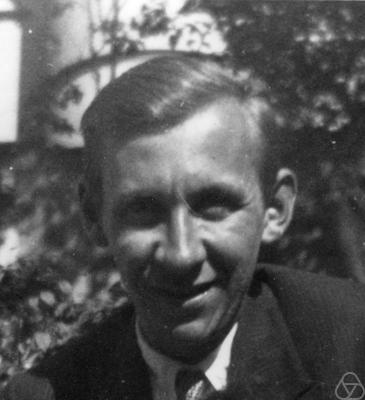# Connections between Cubic and Dual Quadratic Fields

## The Mirror Theorem of Arnold Scholz

Arithmetical Concepts:

Two quadratic number fields k1 and k2 with discriminants d1 and d2 are called dual or a dual pair, if either d1 = -3d2 or d2 = -3d1 (and thus d1 = -d2/3).

One of two dual quadratic fields is real (the field with positive discriminant), the other imaginary. Since discriminants of quadratic fields are essentially squarefree (possibly up to their 2-power contribution), one of two dual discriminants is divisible by 3, the other is not.

The collection of all quadratic number fields is the disjoint union of all dual pairs up to a single exception. A degenerated special case is only the imaginary quadratic field k0 = Q(zeta) with discriminant d = -3, i. e. the cyclotomic field of the 3rd roots of unity zeta = (-1+(-3)1/2)/2 and zeta2 = (-1-(-3)1/2)/2, which is its own dual. Its dual discriminant is formally d' = 1, which does not belong to a quadratic field but to the rational number field Q.

Field Theoretic Background:

By forming the compositum B = k*k', any dual pair (k,k') generates a bicyclic quartic number field B, i. e., a Galois field with automorphism group C(2)xC(2). This elementary Abelian 2-group of rank 2 (KLEIN's four group) comprises exactly three cyclic subgroups. Two of them are mapped onto the intermediate fields k and k' under the GALOIS correspondence, the third subgroup is always mapped onto the cyclotomic field k0 with discriminant d = -3, which forms the mirror axis between k and k' graphically. The situation is illustrated by a field diagram.

The Mirror Theorem of Arnold SCHOLZ:

If we simultaneously apply class field theory  (the ARTIN correspondence between subgroups of the 3-elementary ideal class group of k and unramified cyclic cubic extensions of k, resp. similarly for k')and KUMMER theory  (the KUMMER correspondence between subgroups of the 3-radicand group of k' and cyclic cubic super fields of k, resp. analogously for k and k') to the members k and k' of a duale pair (k,k') and pay attention to some facts concerning the essential ideals [3,5,6] and the 3-primary character of principal ideal cubes [3,4], then we obtain a remarkably simple connection between the 3-class ranks rho and rho' of k and k' which is known as SCHOLZ's mirror theorem  and is illustrated by the following dynamic diagrams.

Remarks concerning the Dynamic Diagrams:

In these diagrams we denote the 3-class ranks shortly by r,r' instead of rho,rho'. In the complex quadratic field k we let a1,...,ar be the principal ideal cubes associated with generating ideal classes of order 3. In the real quadratic field k' we denote by a'1,...,a'r' the principal ideal cubes associated with generating ideal classes, which are joined by the fundamental unit e of k' as a further principal ideal cube. Each of the n(r) = ( 3r - 1 ) / 2 cyclic subgroups of the group < a1,...,ar >, which is isomorphic to the 3-class group of k, generates a cyclic cubic relative extension Ni of k resp. N'i of k' with i = 1,...,n(r), according to class field theory resp. KUMMER theory. And each of the n(r') = ( 3r' - 1 ) / 2 cyclic subgroups of the group < a'1,...,a'r' >, which is isomorphic to the 3-class group of k', generates a cyclic cubic relative extension N'i of k' with i = 1,...,n(r'), according to class field theory. Finally, each of the n(r' + 1) = ( 3r' + 1 - 1 ) / 2 cyclic subgroups of the group < a'1,...,a'r', e > of principal ideal cubes of k' generates a cyclic cubic relative extension Ni of k with i = 1,...,n(r' + 1), according to KUMMER theory. Almost all of these cyclic cubic relative extensions are unramified with conductor f = 1. Only those which depend on the uniquely determined non-primary principal ideal cube among a1,...,ar,a'1,...,a'r',e are ramified with conductor f = 3 or 9.

The case of distinct 3-class ranks in the Mirror Theorem of SCHOLZ, i. e., r = r' + 1, is called escalatory, although it is the most frequent case, statistically . Here, the non-primary principal ideal cube lies in the quadratic field k with negative discriminant d and is characterized by a lower bar. The normal fields of the totally real cubic fields with discriminant d'* = f2 d', where f = 3 for 3 | d' and f = 9 otherwise, that depend on the non-primary principal ideal cube are emphasized by upper bars. In the non escalatory case with identical 3-class ranks r = r', which occurs less frequently, the non-primary principal ideal cube lies in the quadratic field k with positive discriminant d'. I always underbar the fundamental unit e as non-primary, although I have not verified that this is correct and that all the other principal ideal cubes are primary. This question is irrelevant for the structure, however. As before, the normal fields of the complex cubic fields with discriminant d* = f2 d, where f = 3 for 3 | d and f = 9 otherwise, that depend on the non-primary principal ideal cube are emphasized by upper bars.

Bibliography: